## Leafy Vegetables

Leafy Vegetables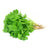Rs. 35.00
Rs. 40.00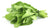Rs. 25.00
Rs. 35.00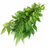Rs. 15.00
Rs. 20.00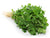Rs. 30.00
Rs. 35.00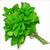Rs. 15.00
Rs. 22.00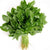Rs. 35.00
Rs. 45.00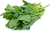Rs. 30.00
Rs. 45.00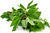Rs. 20.00
Rs. 28.00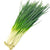Rs. 13.00
Rs. 16.25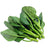Rs. 30.00
Rs. 45.00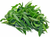Rs. 20.00
Rs. 24.00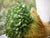Rs. 20.00
Rs. 25.00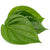Rs. 20.00
Rs. 35.00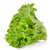Rs. 52.00
Rs. 65.00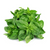Rs. 25.00
Rs. 35.00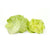Rs. 93.00
Rs. 99.00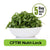Rs. 18.00
Rs. 25.75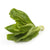Rs. 53.00
Rs. 63.00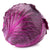Rs. 90.00
Rs. 112.00Rs. 28.00
Rs. 37.50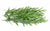Rs. 72.00
Rs. 90.00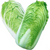Rs. 156.00
Rs. 165.00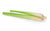Rs. 70.00
Rs. 90.00